In :
import pandas as pd
import numpy as np
import matplotlib.pyplot as plt
import matplotlib
from sqlalchemy import create_engine
engine = create_engine('postgresql://[email protected]:5432/stuartlynn')
%matplotlib inline


# Census segmentation Test¶

The Census produces summary tables of a large number of variables such as number of individuals in a given age bracket, rent range etc. While these are often sufficent for producing segments the joint probabilities for say vision problems by household income are not often avaliable.

Public use microdata however contain the individual responses of a number of individual at PUMA tract levels which allow any conditional probability distribution of any set of variables to be computed.

This document is a test to see if we can train a machine learning model to predict custom probability distributions for a given set of microdata variables and then map these to other census geometries

## Data¶

To get a given breakdown of variables we use the Data Ferret tool provided by the census. This is not ideal for general use but it allows us to perform some easy tests.

From there we extract the variables $DEYE$, $DEAR$ and $age$ brackets

DEYE DEAR
1 Has Vision problem Has Hearing problem
2 No Vision problem No Hearing problem

The datafile pums_age_vision_hearing.csv extracted from Data Ferret has an entry per survey respondant with their age and their responses to the eye and ear questions

In :
data = pd.read_csv("data/pums_age_vision_hearing.csv")

Out:
AGEP DEAR DEYE PUMA10
0 42 2 2 -9
1 42 2 2 -9
2 4 2 2 -9
3 3 2 2 -9
4 58 2 2 -9
In :
fig = plt.figure( figsize= (20,10))
plt.subplot(121)
labels  = ['> 50 problem', '>50 no problem', '<50 problem', '<50 no problem']
ax = data.groupby([data['AGEP'] < 50, 'DEAR']).count()['AGEP'].plot(kind='bar')
ax.set_xticklabels(labels)
ax.set_title("EAR")
plt.subplot(122)
labels  = ['> 50 problem', '>50 no problem', '<50 problem', '<50 no problem']
ax = data.groupby([data['AGEP'] < 50, 'DEYE']).count()['AGEP'].plot(kind='bar')
ax.set_xticklabels(labels)
ax.set_title("EYE")

Out:
<matplotlib.text.Text at 0x5b89c1190>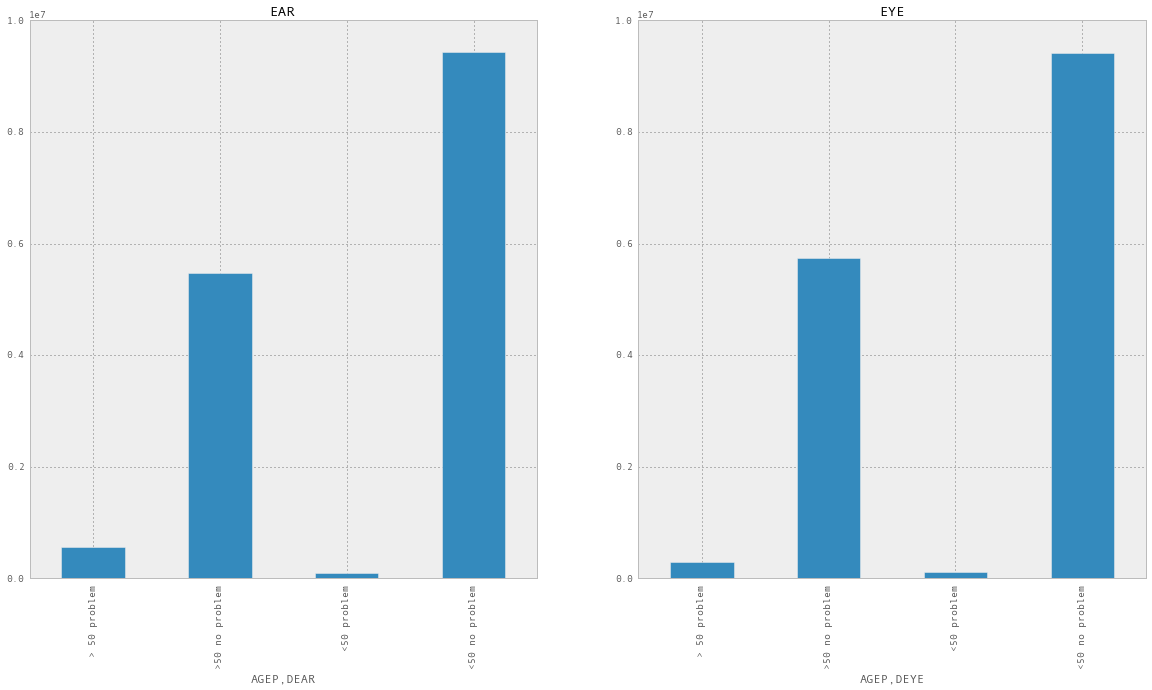We are also going to read in the pumus summary table which contains the same columns as the other census geometries. This will give us the training data we need to train the model

In :
pumas = pd.read_sql_query('select * from pumas_joined', engine)


## Generate the target distribution¶

Our goal is going to be to calculate the joint distribution of a person having eye and ear problems per ceunsus geometry. To do this we group by PUMA and count up the various different variations

In :
eye_ear = data[data['DEYE'] ==1][data['DEAR']==1].groupby("PUMA10").count()['AGEP']
eye_noear = data[data['DEYE']==1][data['DEAR']==2].groupby("PUMA10").count()['AGEP']
noeye_ear = data[data['DEYE']==2][data['DEAR']==1].groupby("PUMA10").count()['AGEP']
noeye_noear = data[data['DEYE']==2][data['DEAR']==2].groupby("PUMA10").count()['AGEP']

summary = pd.DataFrame({ "eye_ear": eye_ear,
"eye_noear": eye_noear,
"noeye_ear": noeye_ear,
"noeye_no_ear":noeye_noear})

Out:
eye_ear eye_noear noeye_ear noeye_no_ear
PUMA10
-9 52160 100221 203987 5817341
100 2221 3774 9436 188686
101 398 924 1786 53645
102 422 955 1920 57291
103 296 734 1266 44842

We can plot out some of the breakdown of our counts across a few PUMAS

In :
summary.head().T.plot(kind='pie', subplots=True, figsize=(80,15))

Out:
array([<matplotlib.axes._subplots.AxesSubplot object at 0x5d1353b50>,
<matplotlib.axes._subplots.AxesSubplot object at 0x6352e28d0>,
<matplotlib.axes._subplots.AxesSubplot object at 0x64f1c5650>,
<matplotlib.axes._subplots.AxesSubplot object at 0x64eb193d0>,
<matplotlib.axes._subplots.AxesSubplot object at 0x10f184050>], dtype=object)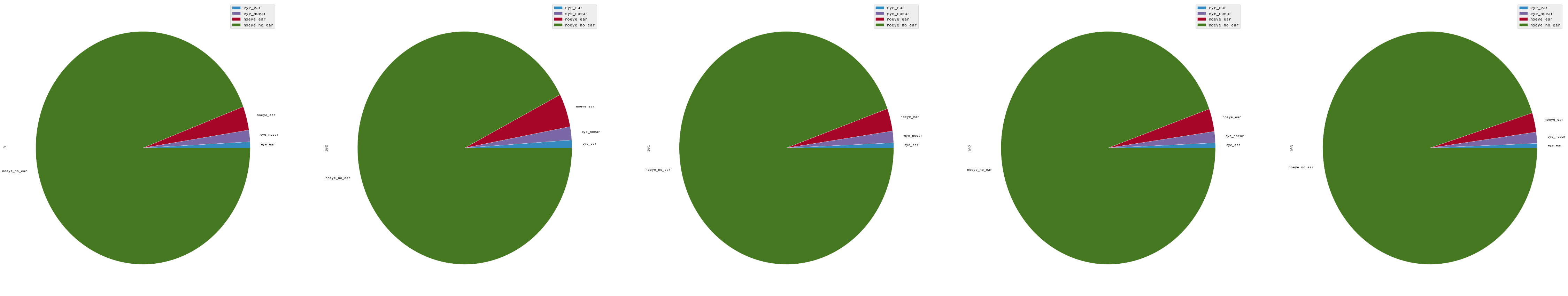## Getting the model features¶

Next step is to generate the model features. We take the PUMAS dataset and discard id's, geometries etc.

In :
a =pumas.set_index( pumas[ 'pumas_10'])
moe_cols  = [ f for f in a.columns if 'moe' in f ]
data  = a[a.columns.difference(['the_geom',
'ogc_fid',
'wkb_geometry',
'cartodb_id',
'geoid',
'pumas_10' ] + moe_cols)].join(summary)


Out:
100_000_to_124_999_in_households 10_000_to_14_999_in_households 10_to_14_years_female_pop 10_to_14_years_male_pop 125_000_to_149_999_in_households 150_000_to_199_999_in_households 15_000_to_19_999_in_households 15_to_17_years_female_pop 15_to_17_years_male_pop 18_and_19_years_female_pop ... total_pop under_5_years_female_pop under_5_years_male_pop vacant_housing_units walked_to_work worked_at_home eye_ear eye_noear noeye_ear noeye_no_ear
pumas_10
109 2126 2678 1607 1616 1256 955 2659 1227 1347 4984 ... 101906 2461 2876 6282 2506 3021 49 83 198 8091
110 9015 1579 7441 7776 7193 10286 1557 4496 4541 2301 ... 205918 6491 6486 3516 2026 5853 54 120 258 9374
111 3986 1151 2645 2622 3676 4024 1634 2109 2044 799 ... 105432 1874 2217 11680 832 5464 29 42 91 2477
803 3036 2648 3765 4242 1582 1486 2309 2214 2288 2438 ... 122624 4044 4452 6364 1055 1132 148 313 571 16515
804 2288 3077 2764 2781 1321 1702 3308 1363 1618 1550 ... 100406 2889 3030 4949 2231 2226 104 194 311 10604

5 rows × 98 columns

We next normalize the various target counts to get a probability matrix across the different variables we are interested in

In :
target= data[summary.columns].apply(lambda x: x/data[summary.columns].sum(axis=1), axis=0)
features = data[data.columns.difference(summary.columns)]
print ' target : ', np.shape(target.as_matrix()), " features", np.shape(features.as_matrix())

 target :  (982, 4)  features (982, 94)

Out:
eye_ear eye_noear noeye_ear noeye_no_ear
pumas_10
109 0.005819 0.009856 0.023513 0.960812
110 0.005507 0.012237 0.026310 0.955945
111 0.010989 0.015915 0.034483 0.938613
803 0.008434 0.017838 0.032541 0.941187
804 0.009275 0.017301 0.027736 0.945688

For the machine learning algorithm to work well we need to make sure that both our features are distributed about 0 and are all scaled to roughtly the same range. to do this we first normalize any columns that are fractional counts by their universe root and then we subtract the mean and divide by the standard deviation.

$$\frac{x- \bar{x}}{ \sigma(x)}$$
In :
def scale_features(features):
housing_brackets  = [ f for f in features if 'in_households' in f and 'gini' not in f]
housing_features = features[housing_brackets].apply(lambda x: x/features[housing_brackets].sum(axis=1))
other_housing = [a for a in  features.columns if 'housing' in a and 'moe' not in a ]
other_housing_features = features[other_housing].apply(lambda x: x/ features[other_housing].sum(axis=1), axis=0)
pop_brackets  = [ f for f in features if 'pop' in f and 'not_a_us_citizen_pop' not in f]

ignore = ['rn','not_a_us_citizen_pop','not_a_us_citizen_pop_moe','total_pop'] + [ r for r in features.columns if 'moe' in r]

scaled_features = features[pop_brackets].apply(lambda x: x/features['total_pop'])
scaled_features = scaled_features.join(housing_features).join(other_housing_features)
normed_features = scaled_features.join(features[features.columns.difference(ignore+housing_brackets+pop_brackets+other_housing)])
normed_features = normed_features[normed_features.columns.difference(['total_pop'])]

return normed_features

def norm_features(features,means,stds):
normed_features = features.apply(lambda x: (x-np.mean(x))/np.std(x), axis=0)
return normed_features

In :
scaled_features  = scale_features(features)
means = scaled_features.apply(lambda x: np.mean(x), axis=0)
stds  = scaled_features.apply(lambda x: np.std(x), axis=0)
normed_features = norm_features(scaled_features,means,stds)

normed_features[['median_age_female',
'median_age',
'percent_household_income_spent_on_rent',
'10_to_14_years_female_pop']].hist( figsize=(20,10))

Out:
array([[<matplotlib.axes._subplots.AxesSubplot object at 0x168d860d0>,
<matplotlib.axes._subplots.AxesSubplot object at 0x168e96f90>],
[<matplotlib.axes._subplots.AxesSubplot object at 0x168ecc890>,
<matplotlib.axes._subplots.AxesSubplot object at 0x1694b59d0>]], dtype=object)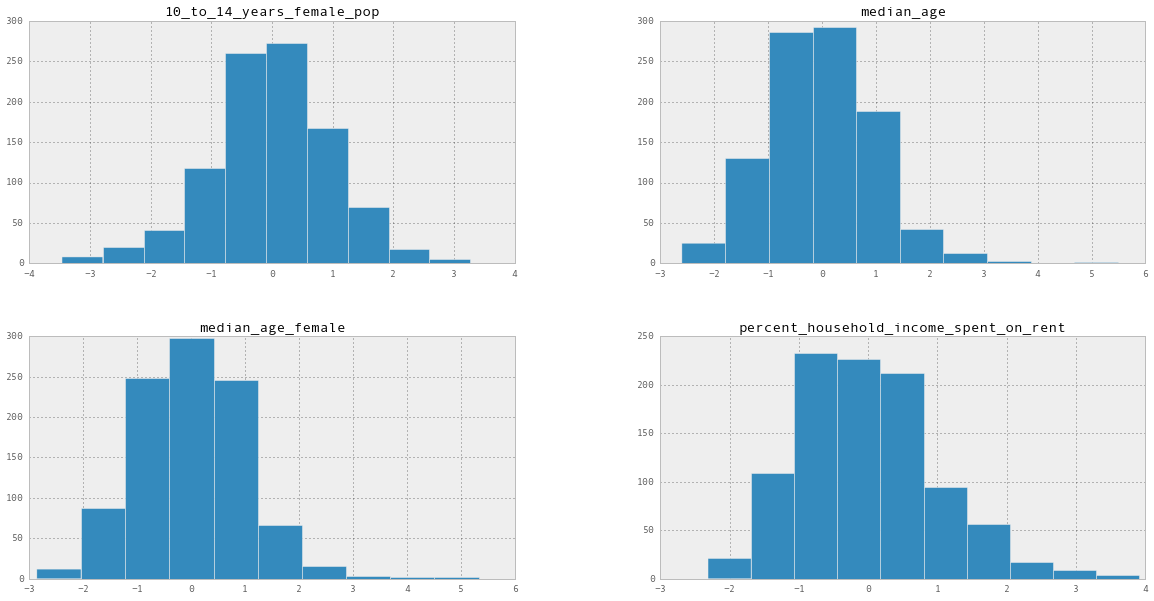In :
scaled_features.to_csv("/Users/stuartlynn/scaled_pumas.csv")

In :
target_means = target.apply(lambda x: np.mean(x), axis=0 )
target_std   = target.apply(lambda x: np.std(x), axis =0)
target_max   = target.apply(lambda x: np.min(x), axis=0)
target_min   = target.apply(lambda x: np.max(x), axis=0)
normed_target = target.apply(lambda x: (x-np.min(x))/(np.max(x)-np.min(x)), axis=0 )
normed_target.hist(figsize=(20,10))

Out:
array([[<matplotlib.axes._subplots.AxesSubplot object at 0x168d303d0>,
<matplotlib.axes._subplots.AxesSubplot object at 0x16c210c90>],
[<matplotlib.axes._subplots.AxesSubplot object at 0x16c29e190>,
<matplotlib.axes._subplots.AxesSubplot object at 0x16c711390>]], dtype=object)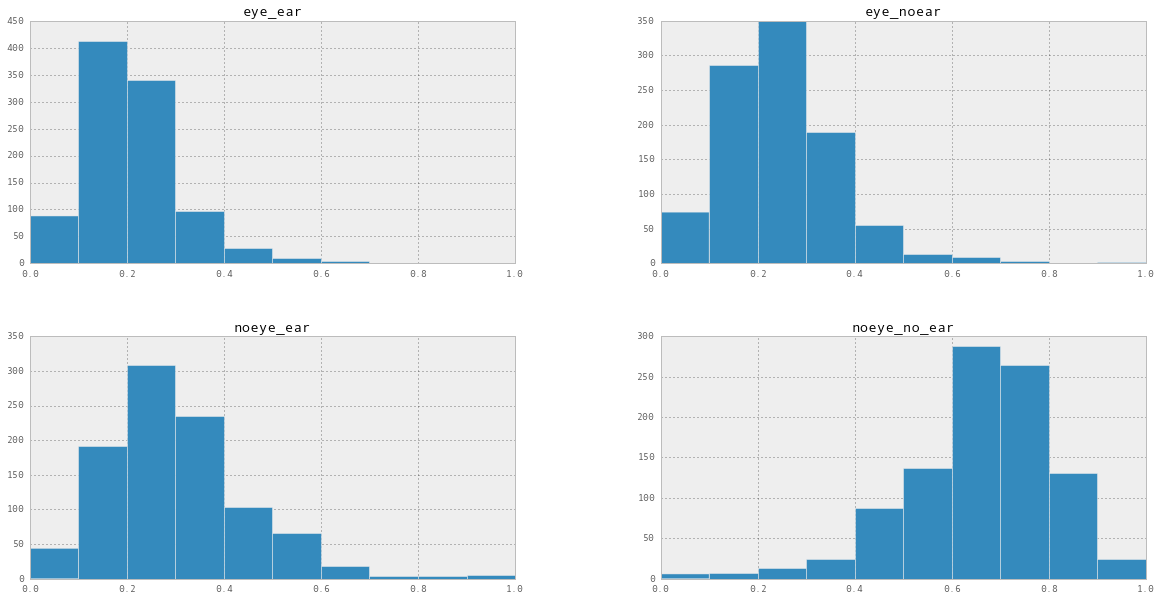## Training the model¶

Now we have the data in good condition we try to train our model. We split the data in to training and test data.

In :
from keras.models import Sequential
from keras.layers.core import Dense, Activation, Dropout
from sklearn.cross_validation  import train_test_split
x_train, x_test, y_train, y_test  = train_test_split(normed_features.as_matrix(),
normed_target.as_matrix(),
test_size=0.30,
random_state=42)

In :
def invert_target(t):
return t*target_std + target_means


Our model is going to be a multiple layer neural network with $tanh$ activation between layers and a linear activation for the final output. We define the loss by the mean squared error and use a stochastic gradient descent method to train the network.

The input dimension is the number of features we are useing and as we are predicting the probability of 4 classes our output layer is a vector or 4 values

In :
model = Sequential()

model.compile(loss='mean_squared_error', optimizer='sgd')


With the model compiled we can fit it to our training set

In :
history = model.fit(x_train, y_train,
verbose=0,
nb_epoch=3000,
batch_size=600,
show_accuracy=True,
validation_data=(x_test, y_test) )

In :
def plot_model_perfromance(history):
fig   = plt.figure(figsize=(20,10))
plt.subplot(121)
plt.title('Loss')
plt.xlabel('epoch')
plt.ylabel('loss')

train = plt.plot(history.epoch, history.history['loss'], 'g-', label='train')
val   = plt.plot(history.epoch, history.history['val_loss'], 'r-', label='validation')
plt.legend( loc='upper left' )
plt.subplot(122)
plt.title('Accuracy')
plt.xlabel('epoch')
plt.ylabel('accuracy')
plt.plot(history.epoch, history.history['acc'], 'g-', label='train')
plt.plot(history.epoch, history.history['val_acc'], 'r-',label='validation')
plt.legend( loc='upper left' )

plot_model_perfromance(history)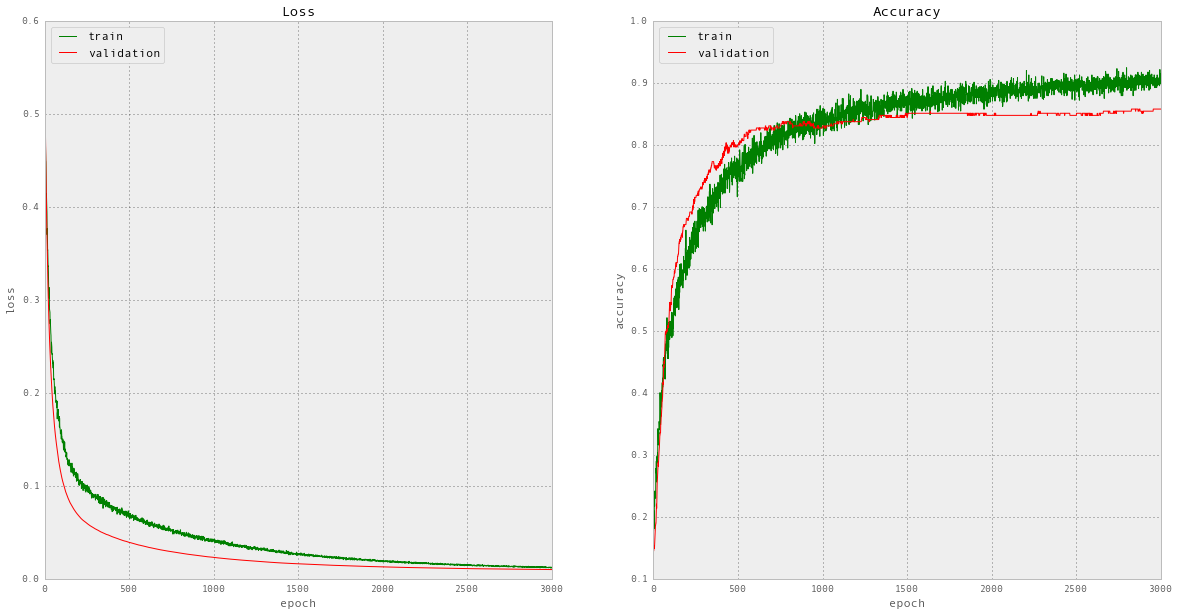In :
score = model.evaluate(x_test, y_test, batch_size=2000)
print 'error %f, error in units of sd %f, accuracy %f ' %(score, score/np.std(y_test), 1-score)

295/295 [==============================] - 0s
error 0.009941, error in units of sd 0.045114, accuracy 0.990059


With the model trained we can predict our test set results and comapre with the actual data

In :
result = model.predict_proba(x_test, batch_size=16)
result

295/295 [==============================] - 0s

Out:
array([[ 0.25860682,  0.33563152,  0.2973173 ,  0.65109867],
[ 0.20303848,  0.14913028,  0.35877565,  0.76830971],
[ 0.20047362,  0.17980911,  0.33872399,  0.79012567],
...,
[ 0.17843936,  0.20258643,  0.28450835,  0.75755018],
[ 0.1778084 ,  0.31786487,  0.32266736,  0.67827946],
[ 0.19241875,  0.32983372,  0.0998479 ,  0.78881645]])
In :
bins = 20
range = [0,1]
alpha = 0.7
fig = plt.figure(figsize=(20,10))
plt.subplot(141)
labels = target.columns

plt.xlabel('Probability')

plt.title("Both vision and hearing disability")
truth      = [r for r in y_test]
prediction = [r for r in result]
range  = [np.min(truth + prediction), np.max(truth + prediction)]
plt.hist(truth, color='red', bins=bins ,alpha =alpha, range=range )
plt.hist(prediction, color='blue', bins=bins, alpha =alpha, range=range )
plt.subplot(142)

plt.title("Vision disability only")
plt.xlabel('Probability')
truth      = [r for r in y_test]
prediction = [r for r in result]
range  = [np.min(truth + prediction), np.max(truth + prediction)]
plt.hist([r for r in y_test], color='red', bins=bins,  alpha =alpha, range=range )
plt.hist([r for r in result], color='blue',bins=bins,  alpha =alpha, range=range )
plt.subplot(143)
plt.title("Hearing disability only")
plt.xlabel('Probability')

truth      = [r for r in y_test]
prediction = [r for r in result]
range  = [np.min(truth + prediction), np.max(truth + prediction)]
plt.hist([r for r in y_test], color='red',bins=bins, alpha =alpha, range=range)
plt.hist([r for r in result], color='blue',bins=bins,  alpha =alpha,range=range )
plt.subplot(144)
plt.title("No disability")
plt.xlabel('Probability')

truth      = [r for r in y_test]
prediction = [r for r in result]
range  = [np.min(truth + prediction), np.max(truth + prediction)]
plt.hist([r for r in y_test], color='red', bins=bins,  alpha =alpha,range=range )
plt.hist([r for r in result], color='blue' ,bins=bins, alpha =alpha,range=range)

Out:
(array([  0.,   1.,   0.,   1.,   0.,   1.,   4.,   8.,  12.,  12.,  16.,
17.,  28.,  41.,  35.,  50.,  34.,  27.,   8.,   0.]),
array([ 0.0864582,  0.1304984,  0.1745386,  0.2185788,  0.262619 ,
0.3066592,  0.3506994,  0.3947396,  0.4387798,  0.48282  ,
0.5268602,  0.5709004,  0.6149406,  0.6589808,  0.703021 ,
0.7470612,  0.7911014,  0.8351416,  0.8791818,  0.923222 ,
0.9672622]),
<a list of 20 Patch objects>)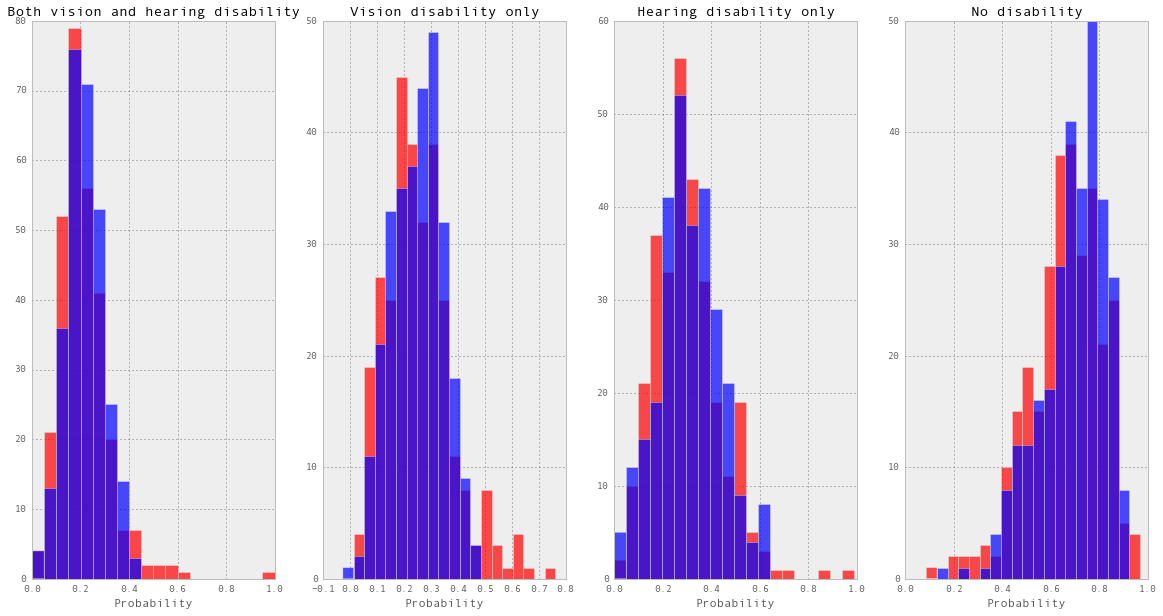In :
fig = plt.figure(figsize=(20,10))

plt.title('Errors')

def invert(x,xmin,xmax):
return x*(xmax-xmin) + xmin

plt.subplot(231)
plt.title("Both vision and hearing disability")
plt.xlabel('Truth')
plt.ylabel('Predction')

truth      = [invert(r,target_min, target_max) for r in y_test]
prediction = [invert(r,target_min, target_max) for r in result]
plt.scatter(truth , prediction)
plt.plot( [np.min(truth), np.max(truth)], [np.min(truth),np.max(truth)], 'r' )

plt.subplot(232)
plt.title("Vision and hearing disability")
plt.xlabel('Truth')
plt.ylabel('Predction')
truth      = [invert(r,target_min, target_max) for r in y_test]
prediction = [invert(r,target_min, target_max) for r in result]
plt.scatter(truth , prediction)
plt.plot( [np.min(truth), np.max(truth)], [np.min(truth),np.max(truth)], 'r' )

plt.subplot(233)
plt.title("Hearing disability only")
plt.xlabel('Truth')
plt.ylabel('Predction')
truth      = [invert(r,target_min, target_max) for r in y_test]
prediction = [invert(r,target_min, target_max) for r in result]
plt.scatter(truth , prediction)
plt.plot( [np.min(truth), np.max(truth)], [np.min(truth),np.max(truth)], 'r' )

plt.subplot(234)
plt.title("No disability")
plt.xlabel('Truth')
plt.ylabel('Predction')

truth      = [invert(r,target_min, target_max) for r in y_test]
prediction = [invert(r,target_min, target_max) for r in result]
plt.scatter(truth , prediction)
plt.plot( [np.min(truth), np.max(truth)], [np.min(truth),np.max(truth)], 'r' )

Out:
[<matplotlib.lines.Line2D at 0x171f97050>]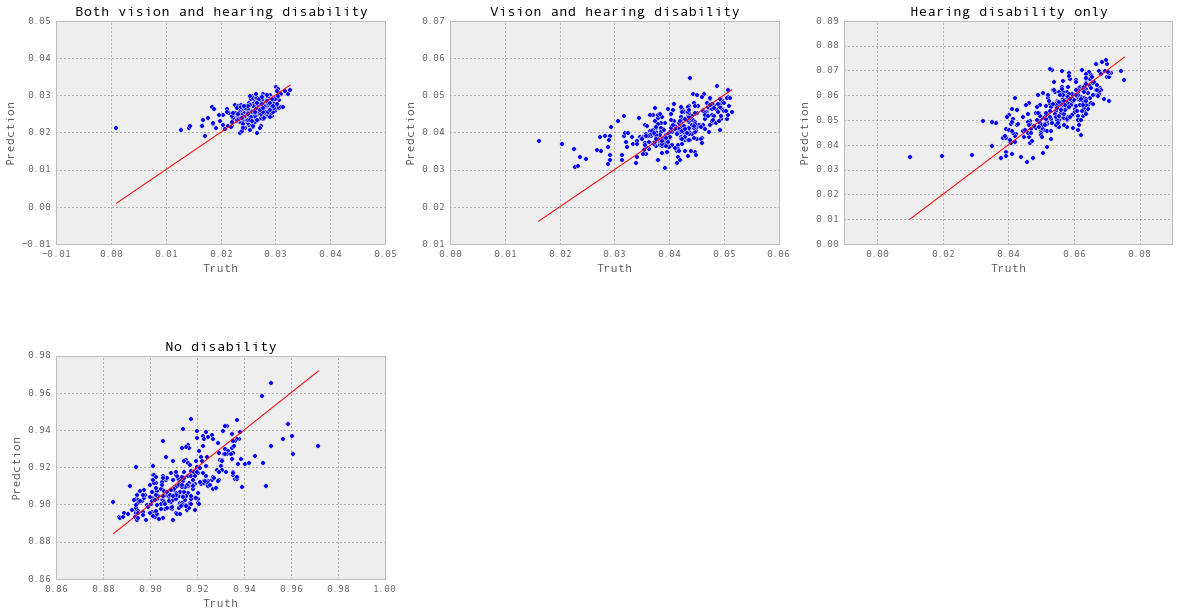## Predicting the output for census blocks¶

In :
feature_list = ','.join(normed_features.columns)
engine,
chunksize=10000)
results = []
geoms   = []

data  = chunk[normed_features.columns | ['total_pop', 'geoid']]
data  = data.convert_objects(convert_numeric=True)
data.index = data['geoid']
new_features = data[data.columns.difference(['geoid'])]

scaled_data = scale_features(new_features)
normed_data = norm_features(scaled_data, means,stds )
prediction = model.predict_proba(normed_data.as_matrix(), batch_size=16)
results.append(pd.DataFrame(prediction,
columns=['eye_ear','eye_noear','noeye_ear', 'noeye_noear'],
index= chunk['geoid'] ))


10000/10000 [==============================] - 0s
10000/10000 [==============================] - 0s
10000/10000 [==============================] - 0s
10000/10000 [==============================] - 1s
10000/10000 [==============================] - 0s
10000/10000 [==============================] - 1s
10000/10000 [==============================] - 0s
4001/4001 [==============================] - 0s

/Users/stuartlynn/anaconda/lib/python2.7/site-packages/IPython/kernel/__main__.py:11: FutureWarning: convert_objects is deprecated.  Use the data-type specific converters pd.to_datetime, pd.to_timedelta and pd.to_numeric.

In :
result = pd.concat(results)
result['eye_ear']= invert(result['eye_ear'], target_min, target_max)
result['eye_noear'] = invert(result['eye_noear'], target_min, target_max)
result['noeye_ear'] = invert(result['noeye_ear'], target_min, target_max)
result['noeye_noear'] = invert(result['noeye_noear'],target_min, target_max)

In :
geom_lookup = pd.read_sql_query('select geoid, the_geom, total_pop from census_tacts_human', engine)
geom_lookup.index= geom_lookup['geoid']

In :
result.join(geom_lookup).to_csv("eye_ear_result.csv")

In :
pumas.index = pumas['pumas_10']
target_with_geom = target.join(pumas[['pumas_10','total_pop','geoid']], )
target_with_geom.to_csv("pumas_eye_ear.csv")


# Results¶

In :
from IPython.display import IFrame


## Origional data on pumas¶

In :
IFrame('https://team.cartodb.com/u/stuartlynn/viz/e02906b6-e0a8-11e5-b257-0e787de82d45/embed_map',
width=800, height=700)

Out:
In :
IFrame('https://team.cartodb.com/u/stuartlynn/viz/355e76f6-e0aa-11e5-bf37-0e5db1731f59/embed_map',
width=800, height=700)

Out:
In [ ]: